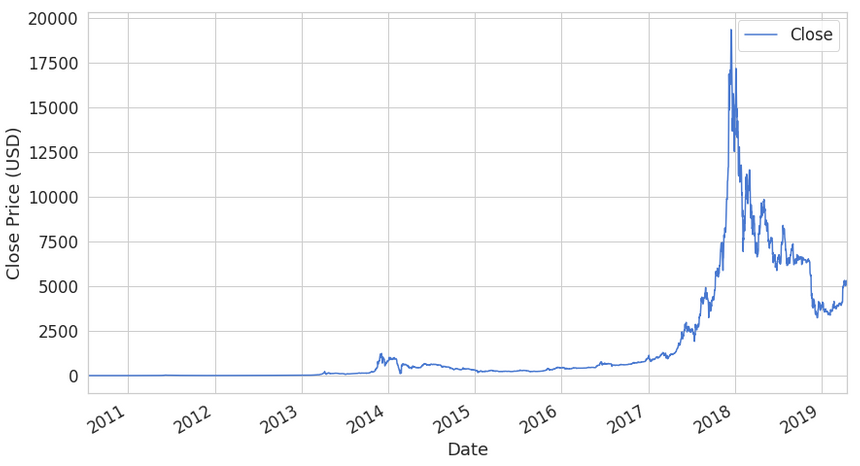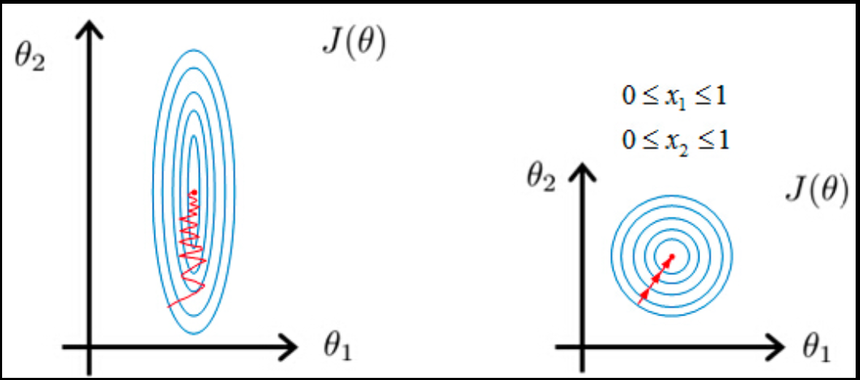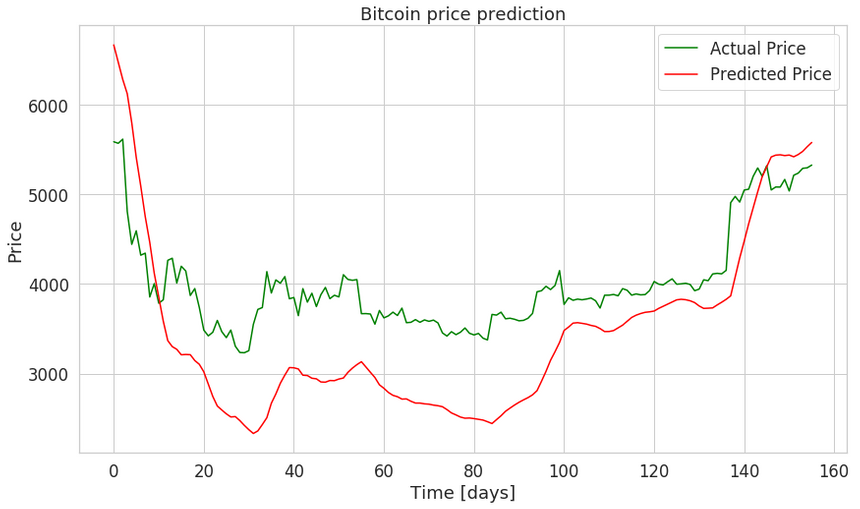# Curiousily

## Cryptocurrency price prediction using LSTMs | TensorFlow for Hackers (Part III)

Deep Learning, Neural Networks, TensorFlow, Python, Time Series, Cryptocurrency4 min read

Share

TL;DR Build and train an Bidirectional LSTM Deep Neural Network for Time Series prediction in TensorFlow 2. Use the model to predict the future Bitcoin price.

Complete source code in Google Colaboratory Notebook

This time you’ll build a basic Deep Neural Network model to predict Bitcoin price based on historical data. You can use the model however you want, but you carry the risk for your actions.

You might be asking yourself something along the lines:

Can I still get rich with cryptocurrency?

Of course, the answer is fairly nuanced. Here, we’ll have a look at how you might build a model to help you along the crazy journey.

Or you might be having money problems? Here is one possible solution:

Here is the plan:

# Data Overview

Our dataset comes from Yahoo! Finance and covers all available (at the time of this writing) data on Bitcoin-USD price. Let’s load it into a Pandas dataframe:

1csv_path = "https://raw.githubusercontent.com/curiousily/Deep-Learning-For-Hackers/master/data/3.stock-prediction/BTC-USD.csv"2df = pd.read_csv(csv_path, parse_dates=['Date'])3df = df.sort_values('Date')

Note that we sort the data by Date just in case. Here is a sample of the data we’re interested in:

DateClose
2010-07-160.04951
2010-07-170.08584
2010-07-180.08080
2010-07-190.07474
2010-07-200.07921

We have a total of 3201 data points representing Bitcoin-USD price for 3201 days (~9 years). We’re interested in predicting the closing price for future dates.Of course, Bitcoin made some people really rich and for some went really poor. The question remains though, will it happen again? Let’s have a look at what one possible model thinks about that. Shall we?

# Time Series

Our dataset is somewhat different from our previous examples. The data is sorted by time and recorded at equal intervals (1 day). Such a sequence of data is called Time Series.

Temporal datasets are quite common in practice. Your energy consumption and expenditure (calories in, calories out), weather changes, stock market, analytics gathered from the users for your product/app and even your (possibly in love) heart produce Time Series.

You might be interested in a plethora of properties regarding your Time Series - stationarity, seasonality and autocorrelation are some of the most well known.

Autocorrelation is the correlation of data points separated by some interval (known as lag).

Seasonality refers to the presence of some cyclical pattern at some interval (no, it doesn’t have to be every spring).

A time series is said to be stationarity if it has constant mean and variance. Also, the covariance is independent of the time.

One obvious question you might ask yourself while watching at Time Series data is: “Does the value of the current time step affects the next one?” a.k.a. Time Series forecasting.

There are many approaches that you can use for this purpose. But we’ll build a Deep Neural Network that does some forecasting for us and use it to predict future Bitcoin price.

# Modeling

All models we’ve built so far do not allow for operating on sequence data. Fortunately, we can use a special class of Neural Network models known as Recurrent Neural Networks (RNNs) just for this purpose. RNNs allow using the output from the model as a new input for the same model. The process can be repeated indefinitely.

One serious limitation of RNNs is the inability of capturing long-term dependencies in a sequence (e.g. Is there a dependency between todays price and that 2 weeks ago?). One way to handle the situation is by using an Long short-term memory (LSTM) variant of RNN.

The default LSTM behavior is remembering information for prolonged periods of time. Let’s see how you can use LSTM in Keras.

## Data preprocessing

First, we’re going to squish our price data in the range [0, 1]. Recall that this will help our optimization algorithm converge faster:source: Andrew Ng

We’re going to use the MinMaxScaler from scikit learn:

1scaler = MinMaxScaler()23close_price = df.Close.values.reshape(-1, 1)45scaled_close = scaler.fit_transform(close_price)

The scaler expects the data to be shaped as (x, y), so we add a dummy dimension using reshape before applying it.

Let’s also remove NaNs since our model won’t be able to handle them well:

1scaled_close = scaled_close[~np.isnan(scaled_close)]2scaled_close = scaled_close.reshape(-1, 1)

We use isnan as a mask to filter out NaN values. Again we reshape the data after removing the NaNs.

### Making sequences

LSTMs expect the data to be in 3 dimensions. We need to split the data into sequences of some preset length. The shape we want to obtain is:

1[batch_size, sequence_length, n_features]

We also want to save some data for testing. Let’s build some sequences:

1SEQ_LEN = 10023def to_sequences(data, seq_len):4    d = []56    for index in range(len(data) - seq_len):7        d.append(data[index: index + seq_len])89    return np.array(d)1011def preprocess(data_raw, seq_len, train_split):1213    data = to_sequences(data_raw, seq_len)1415    num_train = int(train_split * data.shape)1617    X_train = data[:num_train, :-1, :]18    y_train = data[:num_train, -1, :]1920    X_test = data[num_train:, :-1, :]21    y_test = data[num_train:, -1, :]2223    return X_train, y_train, X_test, y_test242526X_train, y_train, X_test, y_test =\27 preprocess(scaled_close, SEQ_LEN, train_split = 0.95)

The process of building sequences works by creating a sequence of a specified length at position 0. Then we shift one position to the right (e.g. 1) and create another sequence. The process is repeated until all possible positions are used.

We save 5% of the data for testing. The datasets look like this:

1X_train.shape
1(2945, 99, 1)
1X_test.shape
1(156, 99, 1)

Our model will use 2945 sequences representing 99 days of Bitcoin price changes each for training. We’re going to predict the price for 156 days in the future (from our model POV).

## Building LSTM model

We’re creating a 3 layer LSTM Recurrent Neural Network. We use Dropout with a rate of 20% to combat overfitting during training:

1DROPOUT = 0.22WINDOW_SIZE = SEQ_LEN - 134model = keras.Sequential()56model.add(Bidirectional(7  CuDNNLSTM(WINDOW_SIZE, return_sequences=True),8  input_shape=(WINDOW_SIZE, X_train.shape[-1])9))10model.add(Dropout(rate=DROPOUT))1112model.add(Bidirectional(13  CuDNNLSTM((WINDOW_SIZE * 2), return_sequences=True)14))15model.add(Dropout(rate=DROPOUT))1617model.add(Bidirectional(18  CuDNNLSTM(WINDOW_SIZE, return_sequences=False)19))2021model.add(Dense(units=1))2223model.add(Activation('linear'))

You might be wondering about what the deal with Bidirectional and CuDNNLSTM is?

Bidirectional RNNs allows you to train on the sequence data in forward and backward (reversed) direction. In practice, this approach works well with LSTMs.

CuDNNLSTM is a “Fast LSTM implementation backed by cuDNN”. Personally, I think it is a good example of leaky abstraction, but it is crazy fast!

Our output layer has a single neuron (predicted Bitcoin price). We use Linear activation function which activation is proportional to the input.

## Training

We’ll use Mean Squared Error as a loss function and Adam optimizer.

1BATCH_SIZE = 6423model.compile(4    loss='mean_squared_error',5    optimizer='adam'6)78history = model.fit(9    X_train,10    y_train,11    epochs=50,12    batch_size=BATCH_SIZE,13    shuffle=False,14    validation_split=0.115)

Note that we do not want to shuffle the training data since we’re using Time Series.

After a lightning-fast training (thanks Google for the free T4 GPUs), we have the following training loss:# Predicting Bitcoin price

Let’s make our model predict Bitcoin prices!

1y_hat = model.predict(X_test)

We can use our scaler to invert the transformation we did so the prices are no longer scaled in the [0, 1] range.

1y_test_inverse = scaler.inverse_transform(y_test)2y_hat_inverse = scaler.inverse_transform(y_hat)`Our rather succinct model seems to do well on the test data. Care to try it on other currencies?

# Conclusion

Congratulations, you just built a Bidirectional LSTM Recurrent Neural Network in TensorFlow 2. Our model (and preprocessing “pipeline”) is pretty generic and can be used for other datasets.

Complete source code in Google Colaboratory Notebook

One interesting direction of future investigation might be analyzing the correlation between different cryptocurrencies and how would that affect the performance of our model.

Share

## Want to be a Machine Learning expert?

Join the weekly newsletter on Data Science, Deep Learning and Machine Learning in your inbox, curated by me! Chosen by 10,000+ Machine Learning practitioners. (There might be some exclusive content, too!)

You'll never get spam from me

© 2021 Curiousily by Venelin Valkov